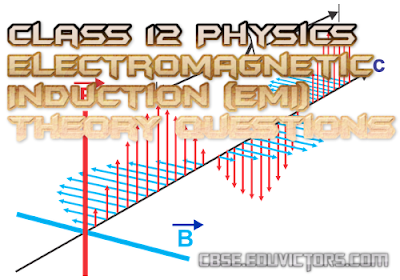## Electromagnetic Induction (EMI)

(Theoretical Questions Assignment)

1. State and Explain Faraday's laws of electromagnetic induction.()

2. State Lenz' Law and show that it is in accordance with the law of conservation of energy.

3. Use Lenz' law to find the direction of induced emf in a coil when
(a) a north pole is brought towards the coil
(b) north pole taken away from the coil
(c) A south pole isbrought towards the coil and
(d) a south pole is taken away from the coil,
Draw illustrations in each case.

4. What is motional emf. Deduce an expression for it. State Fleming's right hand rule to find the direction of induced emf.

5. What are eddy currents? Describe the applications of eddy currents.

6. Explain the working of
(a) Electromagnetic Brakes
(b) Induction Furnace

7. Which physical quantity is called the INERTIA OF ELECTRICITY? Why is its called so?

8. Define self induction and self inductance. What is its unit? Write its dimensions.

9. Derive an expression for the self inductance of a long solenoid.

10. Explain the phenomenon of mutual induction and define mutual inductance. Write the unit and dimensions of mutual inductance.

11. What are the factors affecting mutual inductance of a pair of coils? Define coefficient of coupling.

12. Describe the various methods of producing induced emf. Derive an expression for the instantaneous emf induced in a coil rotated in a magnetic field.

13. What is displacement current? Explain its need.